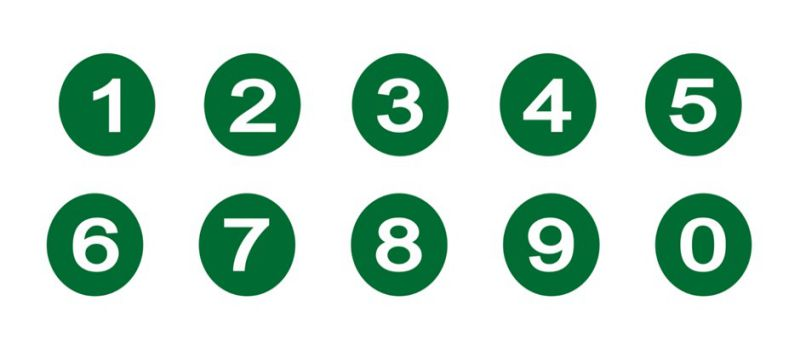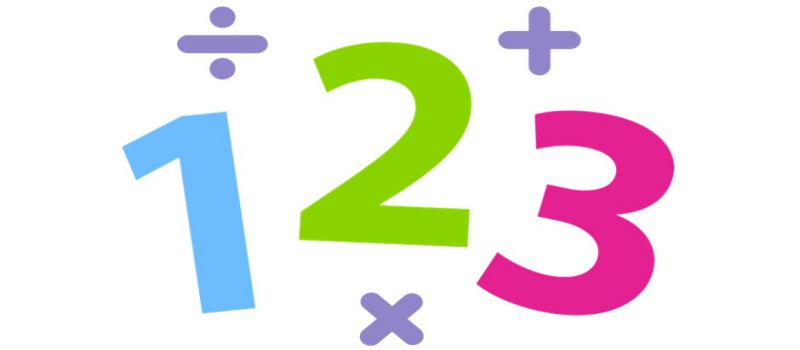Register for Maths, Science, English, Reasoning Olympiad Exams Register here | Book Free Demo for Live Olympiad Classes here | Check Olympiad Exam Dates here | Buy Practice Papers for IMO, IOM, HEO, IOEL etc here | Login here to participate in all India free mock test on every Saturday

# Surprising Maths Short Tricks To Learn | Maths SquareA mathematics wizard has just discovered the largest known prime number and it's an astonishing 17 MILLION digits long.

Curtis Cooper, of the University of Central Missouri, in the US found it by using hundreds of computers that were networked together.

You do not have to be a maths guru to know that a prime is a number greater than 1, which can only be divided by itself and 1, like 2, 3, 5, 7, 11.

However, in the numbers world, size doesn't matter when it comes to the fascinating and bizarre.

Here Peter M Higgins, the author and the numbers man, reveals the magic behind something many of us have struggled to get our heads around.

Hailstone numbersThere are many simple questions about numbers that no one has been able to answer.

Start with any number - if it is even divide it by 2, if odd multiply by 3 and add 1 then keep going, writing down the sequence of numbers that you generate. For example, starting with 7 we are led by these rules through the sequence:

7 -> 22 -> 11 -> 34 -> 17 -> 52 -> 26 -> 13 -> 40 -> 20 -> 10 -> 5 -> 16 -> 8 -> 4 -> 2 -> 1.

It seems no matter what number you start with you eventually hit a 1. These sequences are called the "hailstone numbers" because, like hailstones, they go up and down a number of times before inevitably falling to Earth. However, no one has been able to prove that this has to happen every time.

Twos, threes & fives• Think of a number.
• Add 4, and then multiply the result by 4.
• Subtract 8, and then divide the result by 4.
• Finally take away your original secret number.

Think of another number.

• Double it.
• Divide by 2.

Think of any three-digit number.

• Multiply by 2.
• Subtract 4, and then divide the result by 2.
• Subtract it from the original number you thought of.

Ninety-nine

• Write down any two different numbers from 1 to 9.
• Then reverse the two numbers.
• You should have two two-digit numbers.
• Subtract the smaller number from the larger one.
• Take the result, reverse the digits, and add that number to the one you got when you subtracted.